### Double类型的数据如何保留两位小数？ [问题点数：10分，结帖人foxpeople007]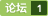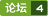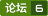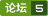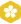double类型保留两位小数
<em>如何</em>将double<em>数据</em>输出为直接截取<em>保留两位小数</em>的字符串？ 解决方法:用BigDecimal类做高精度计算。   Double dd=0.97698; BigDecimal  x=new BigDecimal(dd).setScale(2,BigDecimal.ROUND_DOWN);  String value = String.valueOf(x);  System.out.pri...
int/double类型相除保留两位小数

Java中double类型保留两位小数
java中对<em>double类型</em>的小数经常会有小数点后边保留一位小数或者<em>保留两位小数</em>的需求。 javaAPi中提供了一个专门的类BigDecimal，用来对超过16位有效位的数进行精确的运算。 使用步骤： //定义一个<em>double类型</em>的数字 double number = 3.25; //先将<em>double类型</em>的数字转换为BigDecimal类型。 BigDecimal b

C#中float怎样保留两位小数

Double类型保留2位小数，不要四舍五入

Double 将浮点数值保留两位小数
Double db = new Double(324324.4255);  System.out.println(String.format(&quot;%.2f&quot;, db));
java double类型保留两位小数
package com.sinosoft.demo.controller; import java.math.BigDecimal; import java.text.DecimalFormat; import java.text.NumberFormat; public class Computer { //java中<em>如何</em>使Double类型的数值<em>保留两位小数</em>问题 public
java中Double类型保留两位小数

Double类型保留两位小数并四舍五入
Double类型<em>保留两位小数</em>并四舍五入 package com.utils; import java.math.BigDecimal; import java.math.RoundingMode; import java.text.DecimalFormat; import java.text.NumberFormat; public class DoubleFormatUt...
js中Double类型数据保留两位小数（非四舍五入）
var a = 3.346234325; var b = parseFloat(a).toFixed(3).substring(0,a.length - 1); //这里先将a转换为float类型再保留三位小数，最后截取字符串前a.length - 1位 document.write(b);输出结果应该是3.34;
delphi7 如何使double类型保留两位小数

double total = XXX; String temp=total.ToString("F2"); 当 total是 12,12,32,12.2输出结果是 12.00,12.32,12.20
java double类型保留两位小数4种方法
4种方法，都是四舍五入，例： import java.math.BigDecimal; import java.text.DecimalFormat; import java.text.NumberFormat; public class format { double f = 111231.5585; public void m1
ruby float保留小数的位数
-
double 保留两位小数
double d = 3.1415926; // 四舍五入 double v = new BigDecimal(d).setScale(2, RoundingMode.HALF_UP).doubleValue(); System.out.println(&quot;v=======&quot; + v); v=======3.14 ...
c++里面如何保留两位小数
-
java double类型实现保留两位小数的方法

double类型数据小数点后面位数太长，怎么样才能保留两位小数呀？
<em>double类型</em>的<em>数据</em>小数点后面位数太长，怎么样才能<em>保留两位小数</em>呀？
springboot对Double类型保留两位小数返回处理

1.<em>数据</em>库字段类型 查出的<em>数据</em>小数点后带两位小数 2.后台查出的json却没有两位小数 [{ recordId : 20170320142740038015948, appSysId : 23551, appDeptId : 3, orgDeptName : null, carHostNO : 1019, secureNo : 111, sec
double类型数据............

qt 怎么保留两位整数?
-
JS 计算的结果 保留两位小数
function getA(obj){ var id = obj.id; var pre = id.spli
c#字符串类型小数能直接保留2位小数吗，谢谢
-
c# 让double保留两位小数
1、Math.Round(0.333333,2);//按照四舍五入的国际标准 2、 double dbdata=0.335333; string str1=String.Format(&quot;{0:F}&quot;,dbdata);//默认为保留两位 还有一个类似的方法，但是不提倡： double d1 = 0.335333; string d2 = d1.ToString(&quot;...
decimal类型保留两位小数，并保持decimal类型

java中如何使Double类型的数值保留两位小数问题

#includernint main()rnrn float b = 2345.23456;rn b=int(b*100)/100.0;rn printf("%f", b);rn return 0;rnrn为何我后面精度有问题，请问有人知道怎么解决吗？

double保留小数点后两位的问题，跪求大神详解
-
c#中double类型如何保留小数点后2位。

C#中的decimal如何保留两位小数？？？

<em>数据</em>强制<em>保留两位小数</em>，记录分享：前台写法：首先用到EL表达式时这样处理a、引入标签库&amp;lt;%@ taglib prefix=&quot;c&quot; uri=&quot;http://java.sun.com/jsp/jstl/core&quot; %&amp;gt; &amp;lt;%@ taglib prefix=&quot;fmt&quot; uri=&quot;http://java.sun.com/jsp/jstl/fmt&quot; %&amp;gt; 使用&amp;lt;fmt:f

@JsonSerialize输出数据保留两位小数

sscanf不能读double类型数据
double x,y,z;rnsscanf("123 456 789", "%e %e %e", &x, &y, &z);rn//orrn//sscanf("123 456 789", "%f %f %f", &x, &y, &z);rnrn以上代码不能正确的读取<em>double类型</em>的<em>数据</em>，请问<em>如何</em>解决？rn谢谢！
double类型数据(返回类型也是double保留小数部分2位)
<em>double类型</em>的<em>数据</em>保留小数部分2位,返回的是<em>double类型</em>也要是2位,而且位数不足会补零 例如: int m=3,n=19; double result=(double)m/n; Decimal
Double 类型保留两位小数

JAVA笔记:double四舍五入并保留两位小数的方法
1.只要输出结果 1 2 double x1 = 0.026; System.out.println(String.format(&quot;%.2f&quot;, x1));     2.<em>数据</em>转换 1 2 3 4 5 6 7 8 9 10 11 ...
js如何保留两位小数

double类型数据的问题

double类型数据格式化
DecimalFormat df = new DecimalFormat(&quot;0.00&quot;); double d = 123.9078; String db = df.format(d); System.out.println(db); //123.91 会四舍五入
Double类型数据显示格式处理
<em>double类型</em><em>数据</em>后面为0则不显示 private String datacheck(double num){            if(num % 1.0 == 0){                    return String.valueOf((long)num);            }            return String.valueOf(num);    }...
double类型数据相除问题？

double数据类型保留两位小数的乘以100后小数点后一长串

double 保留2位小数
package com.woozoom.agrigcs.utils; import java.math.BigDecimal; /** * Created by Administrator on 2017/11/15. */ public class DoubleUtils { //默认除法运算精度 private static final int DEF_DIV_SC
double类型数据保留2位小数
//使用 DecimalFormat 类（import java.text.DecimalFormat;） double o = (10+11*0.8+14*0.5)/87*100; System.out.println(new DecimalFormat(&quot;.##&quot;).format(o)+&quot;%&quot;); 输出：29.66%
Java double类型数据四则运算
import java.math.BigDecimal;public class DoubleArith { public static double add(double augend, double addend) { BigDecimal augendDecimal = new BigDecimal(Double.toString(augend)); Bi
double类型数据显示
double fNum=1234567890;rncout<
double类型数据怎样截取
<em>double类型</em>的<em>数据</em>怎样截取rndouble n=3.254rndouble m=6.258rn我想把任何<em>double类型</em>的<em>数据</em>都截取为只有两位，并且都要进一，不管是否为四舍五入。rn这样rnn=3.26rnm=6.26
double类型，精确的数据运算
<em>double类型</em>下用于精确计算的类包，可自己确定精确位数。复制到项目中即可使用
java double类型数据保留小数位的几个方法

double保留两位小数的多方法
java的double<em>保留两位小数</em>的多方法
string 转换成double，小数点后只有6位
-
double类型数据初始化

double类型数据除以double类型数据 怎么保留小数点后两位
<em>double类型</em><em>数据</em>除以<em>double类型</em><em>数据</em> 怎么保留小数点后两位

<em>如何</em>让查询出来的<em>数据</em>只<em>保留两位小数</em>rn表A:rnA Brn1 12.345rn表BrnA B Crn1 12.345 12.35rnC=A*B

double保留两位小数

Java中保留两位小数，和kotlin保留两位小数
Java   使用BigDecimal，保留小数点后两位 public static String format1(double value) { BigDecimal bd = new BigDecimal(value); bd = bd.setScale(2, RoundingMode.HALF_UP); return bd.toString(); } 使用...
Java使double保留两位小数的多方法 Java保留两位小数
import java.text.DecimalFormat; DecimalFormat df = new DecimalFormat("#.00"); double d1 = 1.23456 double d2 = 2.0; double d3 = 0.0; system.out.println(df.format(d1),df.format(d2),df.format(d3

1、题目： 题目描述 数列的第一项为n，以后各项为前一项的平方根，求数列的前m项的和。 输入描述: 输入<em>数据</em>有多组，每组占一行，由两个整数n（n 输出描述: 对于每组输入<em>数据</em>，输出该数列的和，每个测试实例占一行，要求精度保留2位小数。 示例1 输入 81 4 2 2 输出 94.73 3.41

Scala double保留小数的方法
scala使用formatted可设置保留小数的位数，采用四舍五入 scala&amp;gt; val a=12.34562 a: Double = 12.34562 scala&amp;gt; a.formatted(&quot;%.2f&quot;) #其中2表示保留小数位数 res3: String = 12.35 scala&amp;gt; a.formatted(&quot;%.4f&quot;) res4: String = 12.34...
Java 将String转Double、Double类型保留2位小数

Jackson转换实体 double 保留两位小数

android保留double小数后两位的方法
android中使用TextView或者EditText是保留double小数后两位的方法： // import java.text.DecimalFormat; // 使用格式化，四舍五入仅保留有效位 // DecimalFormat df = new DecimalFormat("#.##"); // 使用格式化，四舍五入，不足位补零 DecimalFormat df = new Decimal

<em>保留两位小数</em> Codevs天梯 题目描述 Description <em>保留两位小数</em>输出一个浮点数。 输入描述 Input Description 一个浮点数。double范围内 输出描述 Output Description <em>保留两位小数</em>输出 样例输入 Sample Input 11 样例输出 Sample Output 11.00 Code: #include &amp;lt;bits/stdc++.h&amp;g...